# Candlestick chart in R with quantmod

Joshua Ulrich

## The `chartSeries` function

The `quantmod` package allows obtaining, transforming and plotting financial data from different sources. In the following example we are downloading the SP500 data from Yahoo Finance and plotting it with the `chartSeries` function.

Note that you can transform the daily data into weekly or monthly candles with `as.weekly` and `as.monthly` functions, e.g. `as.monthly(GSPC)`.

Default candlestick chart

``````# install.packages("quantmod")
library(quantmod)

# Dates
start <- "2020-10-01"
end <- "2021-01-01"

# Get the data
getSymbols("^GSPC",
from = start, to = end,
src = "yahoo")

# Plot the data
chartSeries(GSPC)``````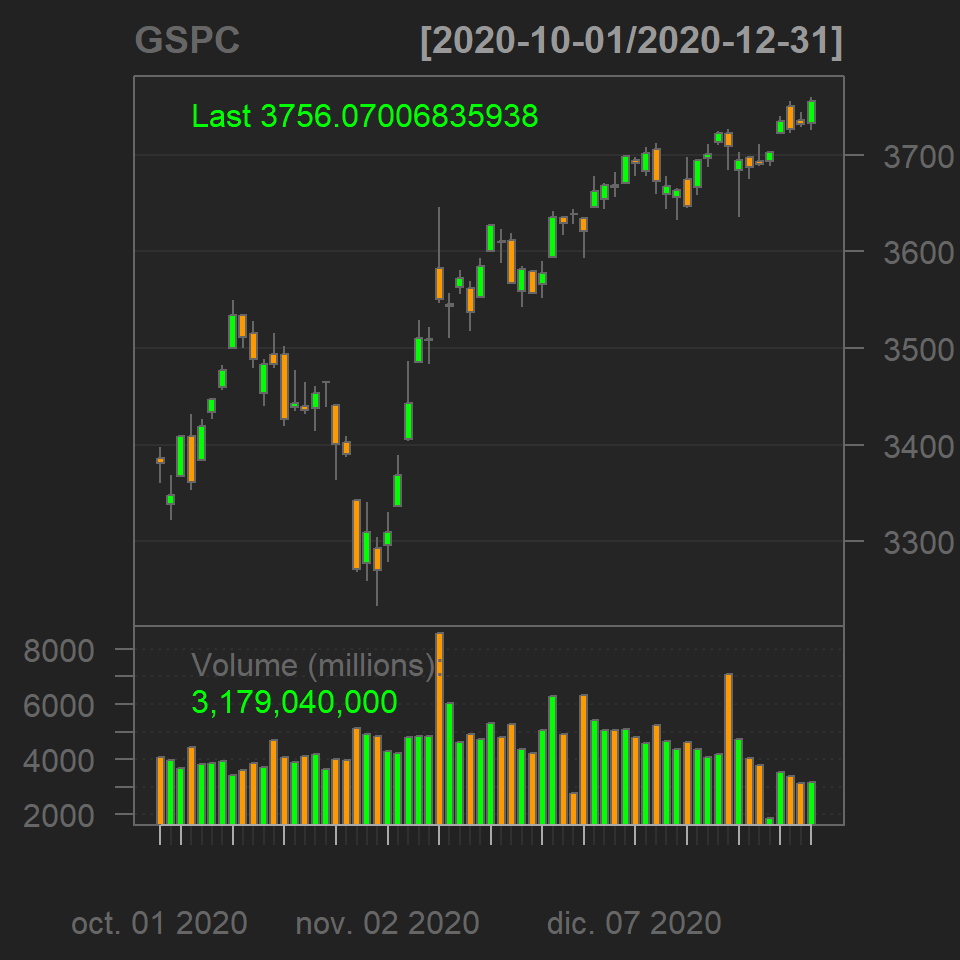The function provides theming and styling options, such as a white theme, changing the bar type, the colors of the candles among others. Type `?chartSeries` for additional options.

Styling

``````# install.packages("quantmod")
library(quantmod)

# Dates
start <- "2020-10-01"
end <- "2021-01-01"

# Get the data
getSymbols("^GSPC",
from = start, to = end,
src = "yahoo")

# Plot the data
chartSeries(GSPC,
theme = chartTheme("white"), # Theme
bar.type = "hlc",  # High low close
up.col = "green",  # Up candle color
dn.col = "pink")   # Down candle color``````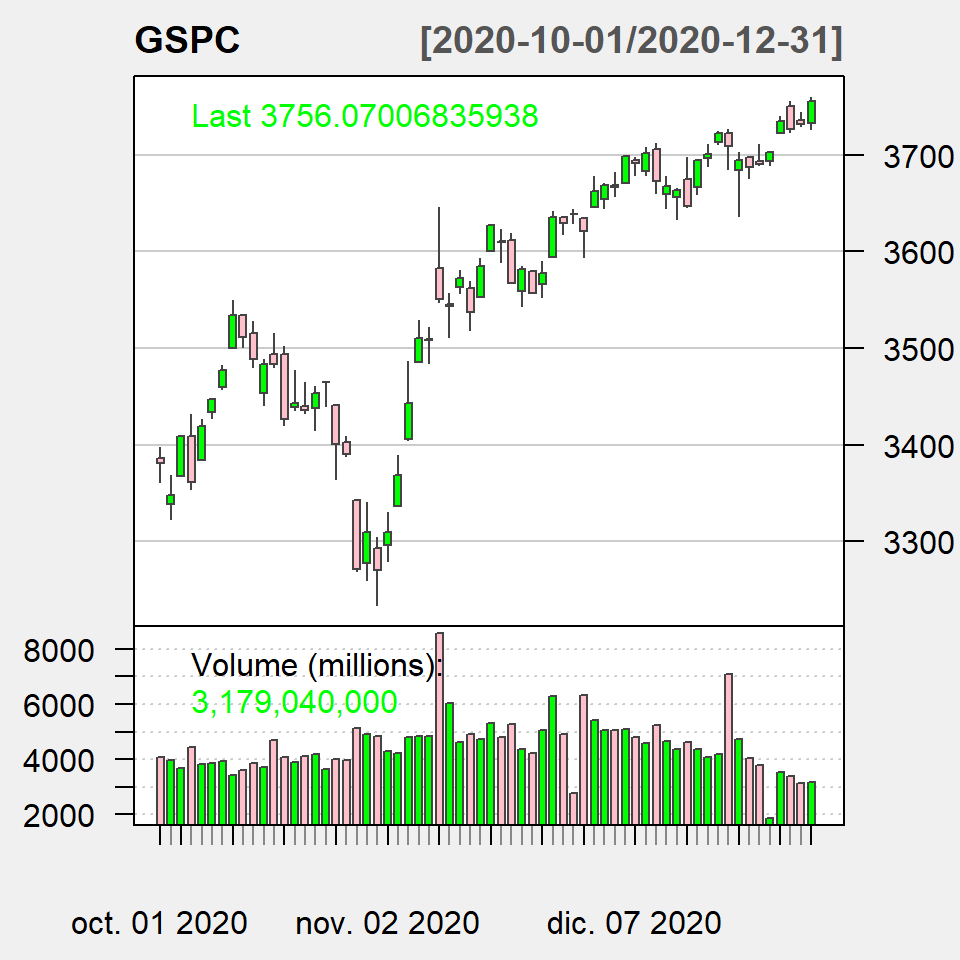You can also add technical indicators to the chart, such as Bollinger bands or the exponential moving average, among others. Look for the functions that starts with `add`.

Technical indicators

``````# install.packages("quantmod")
library(quantmod)

# Dates
start <- "2020-10-01"
end <- "2021-01-01"

# Get the data
getSymbols("^GSPC",
from = start, to = end,
src = "yahoo")

# Plot the data
chartSeries(GSPC,
theme = chartTheme("white"),
name = "SP500",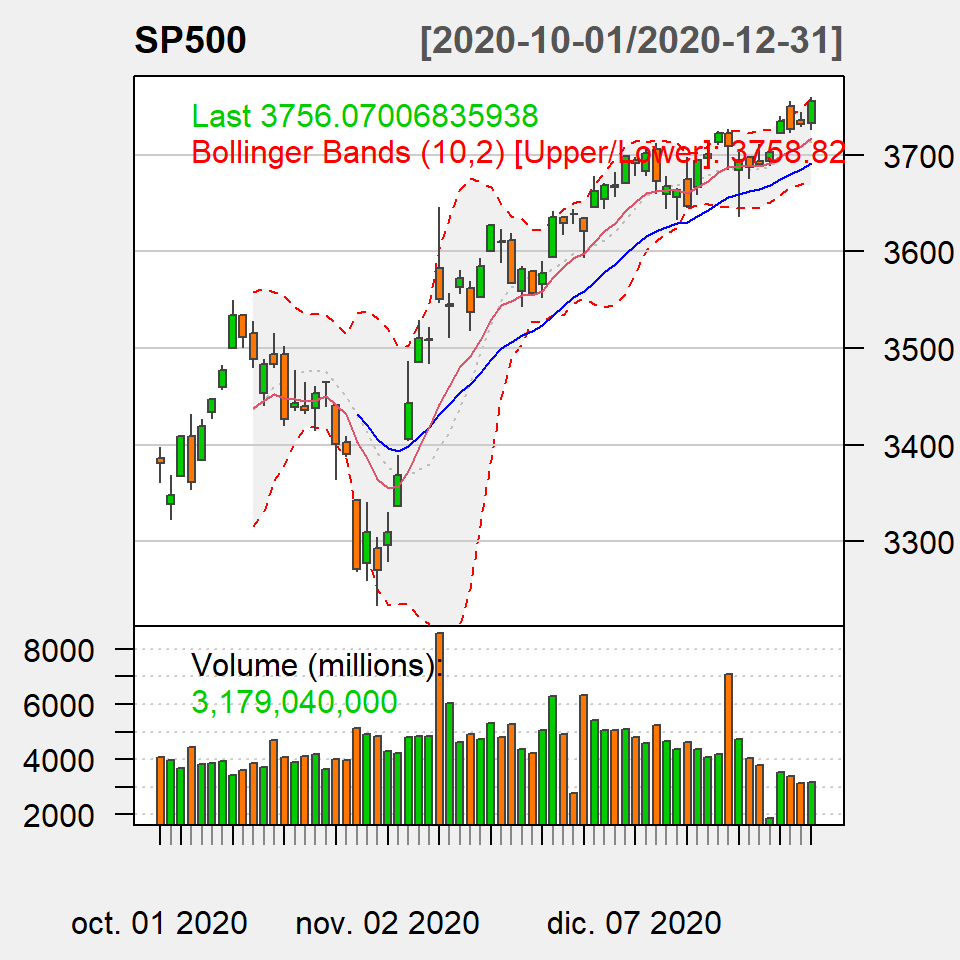## The `chart_Series` function

The `quantmod` package has several experimental functions, such as `chart_Series`. If you pass the financial data this function the default chart is the following: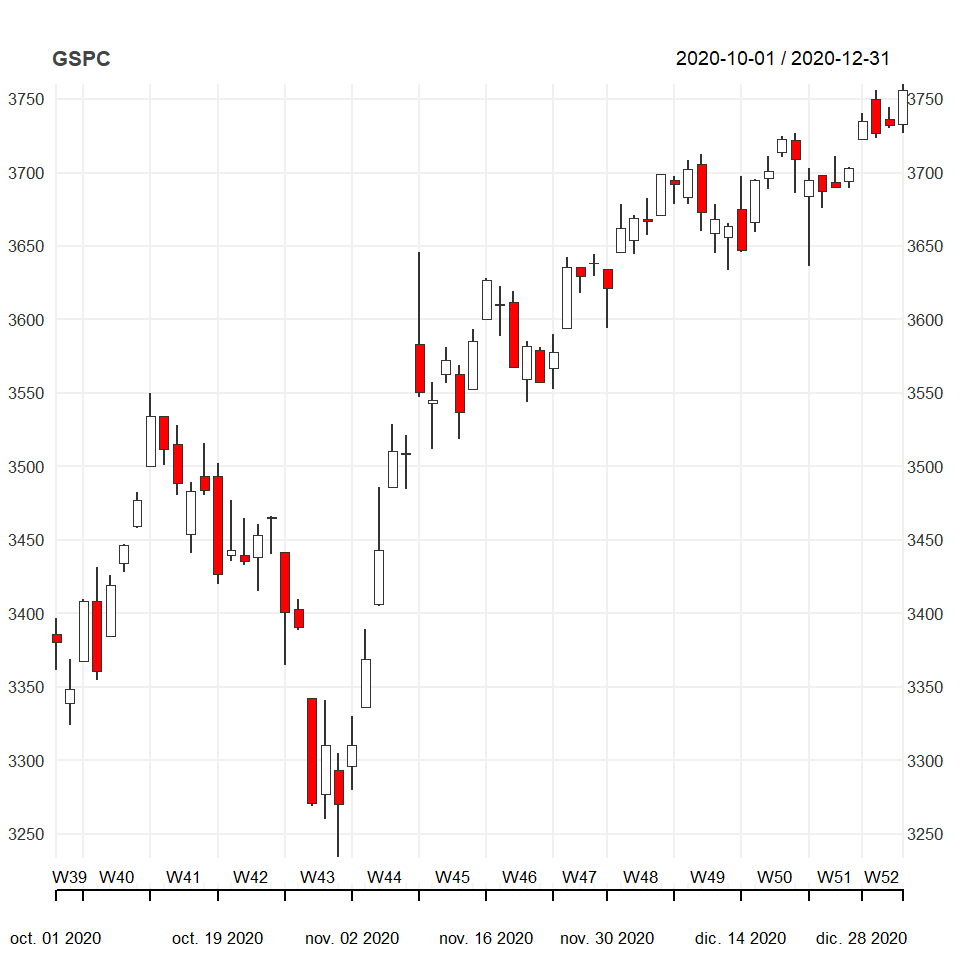``````# install.packages("quantmod")
library(quantmod)

# Dates
start <- "2020-10-01"
end <- "2021-01-01"

# Get the data
getSymbols("^GSPC",
from = start, to = end,
src = "yahoo")

# Plot the data
chart_Series(GSPC)``````

The theme of the chart can be customized with the list of arguments provided inside `chart_theme`.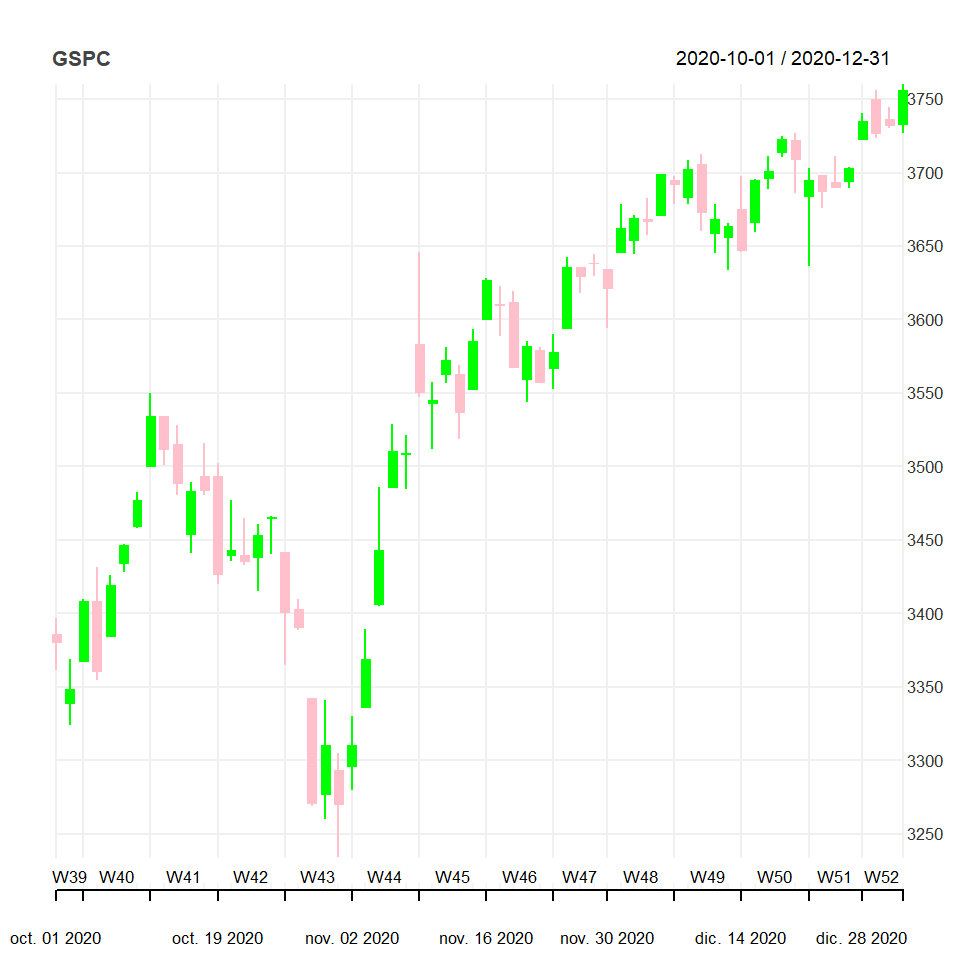``````# install.packages("quantmod")
library(quantmod)

# Dates
start <- "2020-10-01"
end <- "2021-01-01"

# Get the data
getSymbols("^GSPC",
from = start, to = end,
src = "yahoo"

# Custom theme
myTheme <- chart_theme()
myTheme\$col\$dn.col <- "pink"
myTheme\$col\$dn.border <- "pink"
myTheme\$col\$up.col <- "green"
myTheme\$col\$up.border <- "green"
myTheme\$rylab <- TRUE
myTheme\$lylab <- FALSE

# Plot the data
chart_Series(GSPC, theme = myTheme)``````

Finally, you can add technical indicators the same way as in the function of the first section.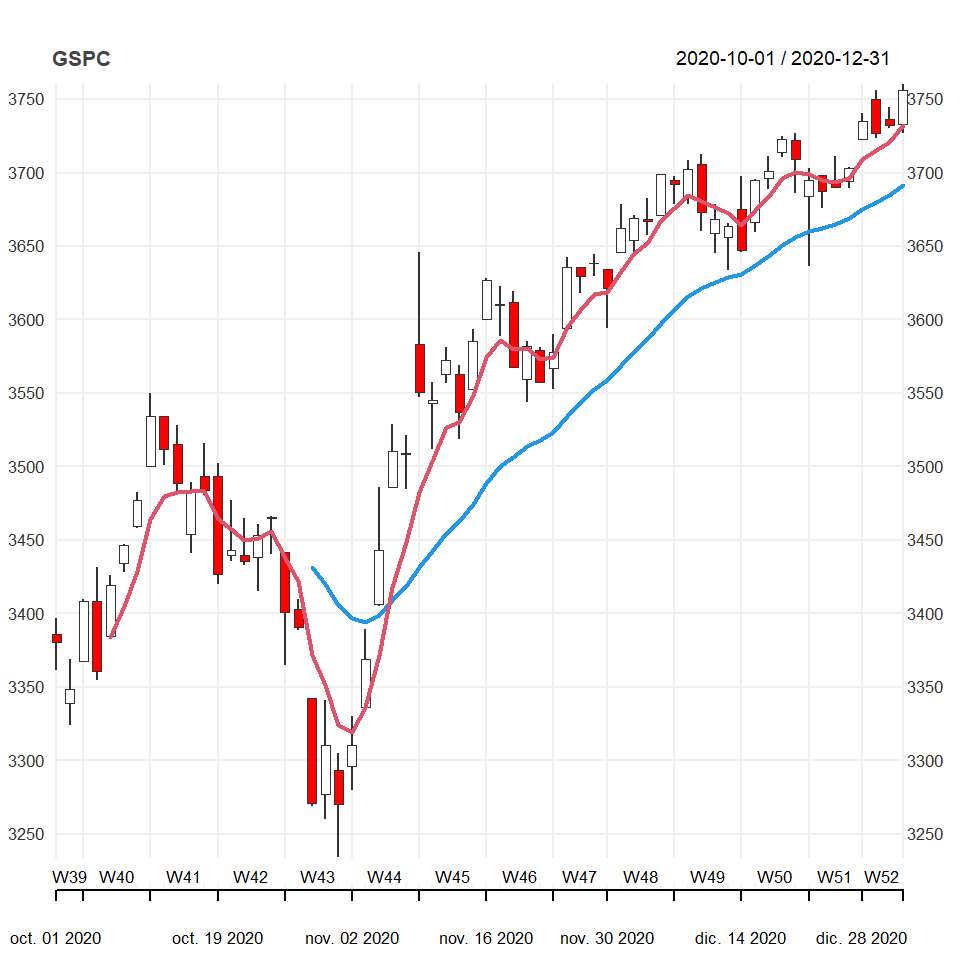``````# install.packages("quantmod")
library(quantmod)

# Dates
start <- "2020-10-01"
end <- "2021-01-01"

# Get the data
getSymbols("^GSPC",
from = start, to = end,
src = "yahoo")

# Plot the data
chart_Series(GSPC,
TA = list("add_EMA(n = 20, col = 4,
lwd = 2)",
"add_EMA(n = 5, col = 2,
lwd = 2)"))``````